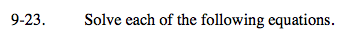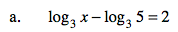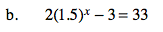### Home > PCT > Chapter Ch9 > Lesson 9.1.2 > Problem9-23

9-23.
1. Solve each of the following equations. Homework Help ✎

1. log3 x − log3 5 = 2

2. 2(1.5)x − 3 = 33$\text{log}_{3} \frac{x}{5}= 2$

$3^2=\frac{x}{5}$